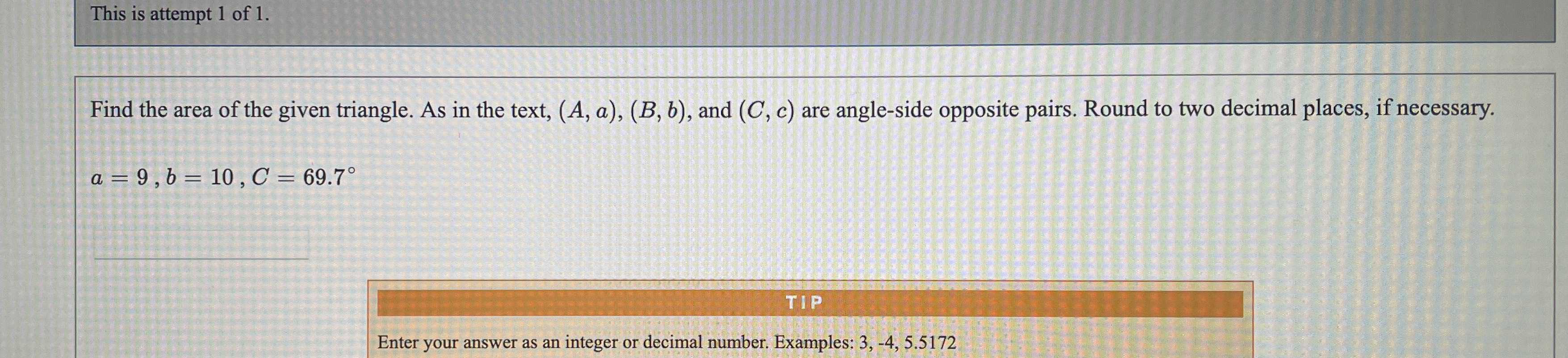### Still have math questions?

Trigonometry
QuestionFind the area of the given triangle. As in the text, $$( A , a ) , ( B , b )$$ , and $$( C , c )$$ are angle-side opposite pairs. Round to two decimal places, if necessary.

$$a = 9 , b = 10 , C = 69.7 ^ { \circ }$$

Enter your answer as an integer or decimal number. Examples: $$3 , - 4,5.5172$$